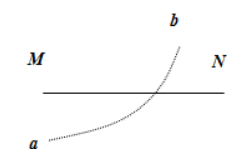$\text{A.}$ 带电粒子从 $a$ 到 $b$ 运动的过程中动能逐渐减小 $\text{B.}$ 带电粒子在 $a$ 点时的电势能大于在 $b$ 点时的电势能 $\text{C.}$ 带电粒子在 $a$ 点时的加速度大于在 $b$ 点时的加速度 $\text{D.}$ 负点电荷一定位于 $N$ 点右侧

AC

#### 解析：

$B$ : 电场力对带电粒子做负功, 电势能增大, 则带电粒子在 $a$ 点的电势能小于在 $b$ 点的电势能, 故 $B$ 错误；
$C D$ : 由上得知, 带正电粒子的电场力向左, 电场线由 $N$ 指向 $M$, 说明负电荷在直线 $M N$ 左侧, $a$ 点离点电荷较近, $a$ 点的电场 强度大于 $b$ 点的电场强度, 根据牛顿第二定律得知, 带电粒子在 $a$ 点的加速度大于在 $b$ 点的加速度。故 $C$ 正确, $D$ 错。